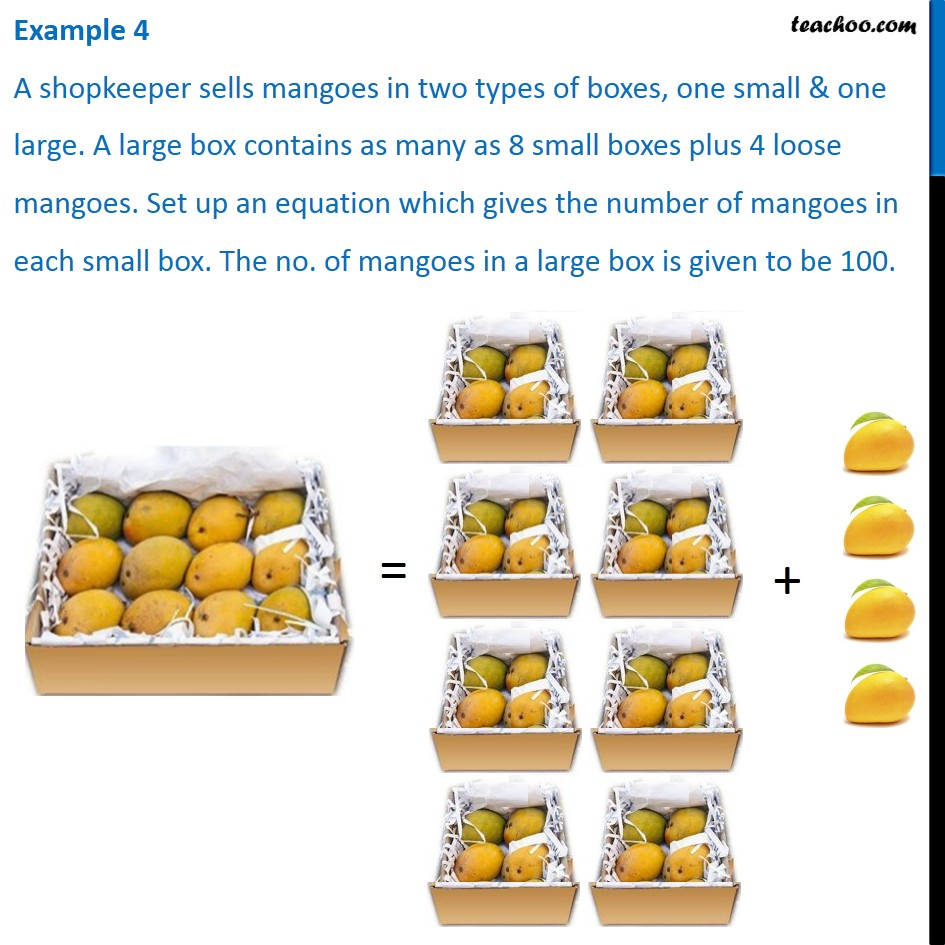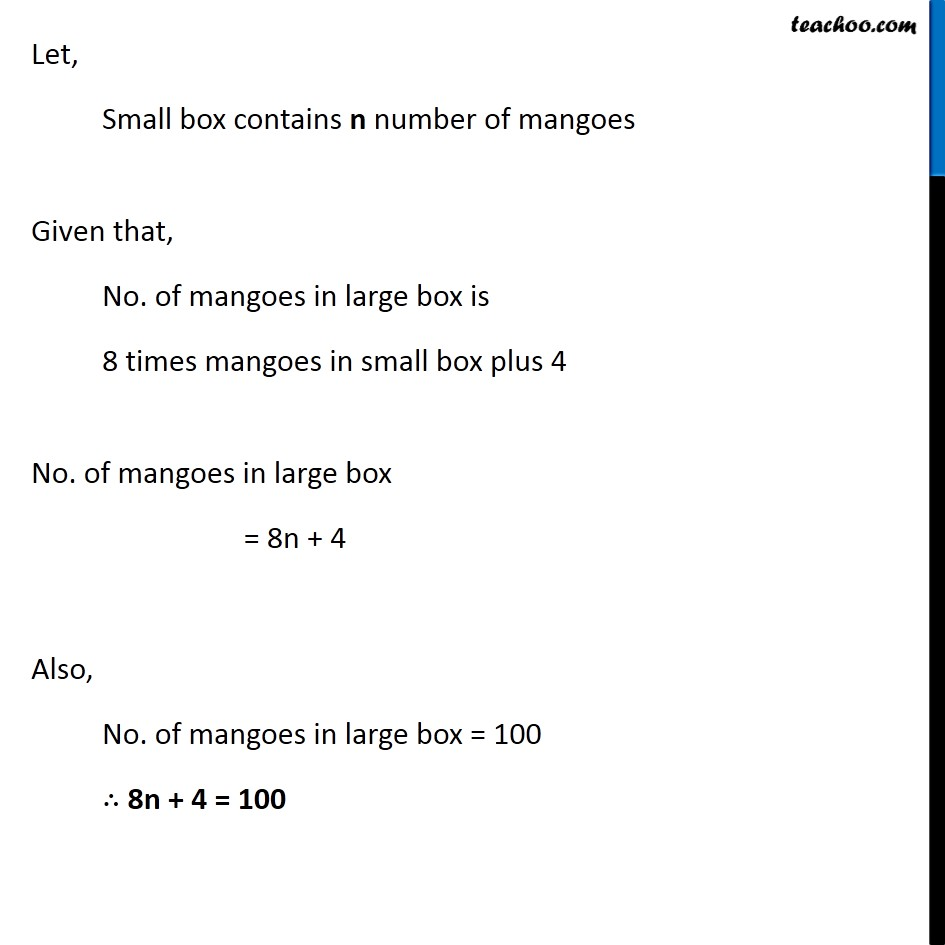1. Chapter 4 Class 7 Simple Equations
2. Serial order wise
3. Examples

Transcript

Example 4 A shopkeeper sells mangoes in two types of boxes, one small & one large. A large box contains as many as 8 small boxes plus 4 loose mangoes. Set up an equation which gives the number of mangoes in each small box. The no. of mangoes in a large box is given to be 100. Let, Small box contains n number of mangoes Given that, No. of mangoes in large box is 8 times mangoes in small box plus 4 No. of mangoes in large box = 8n + 4 Also, No. of mangoes in large box = 100 ∴ 8n + 4 = 100

Examples